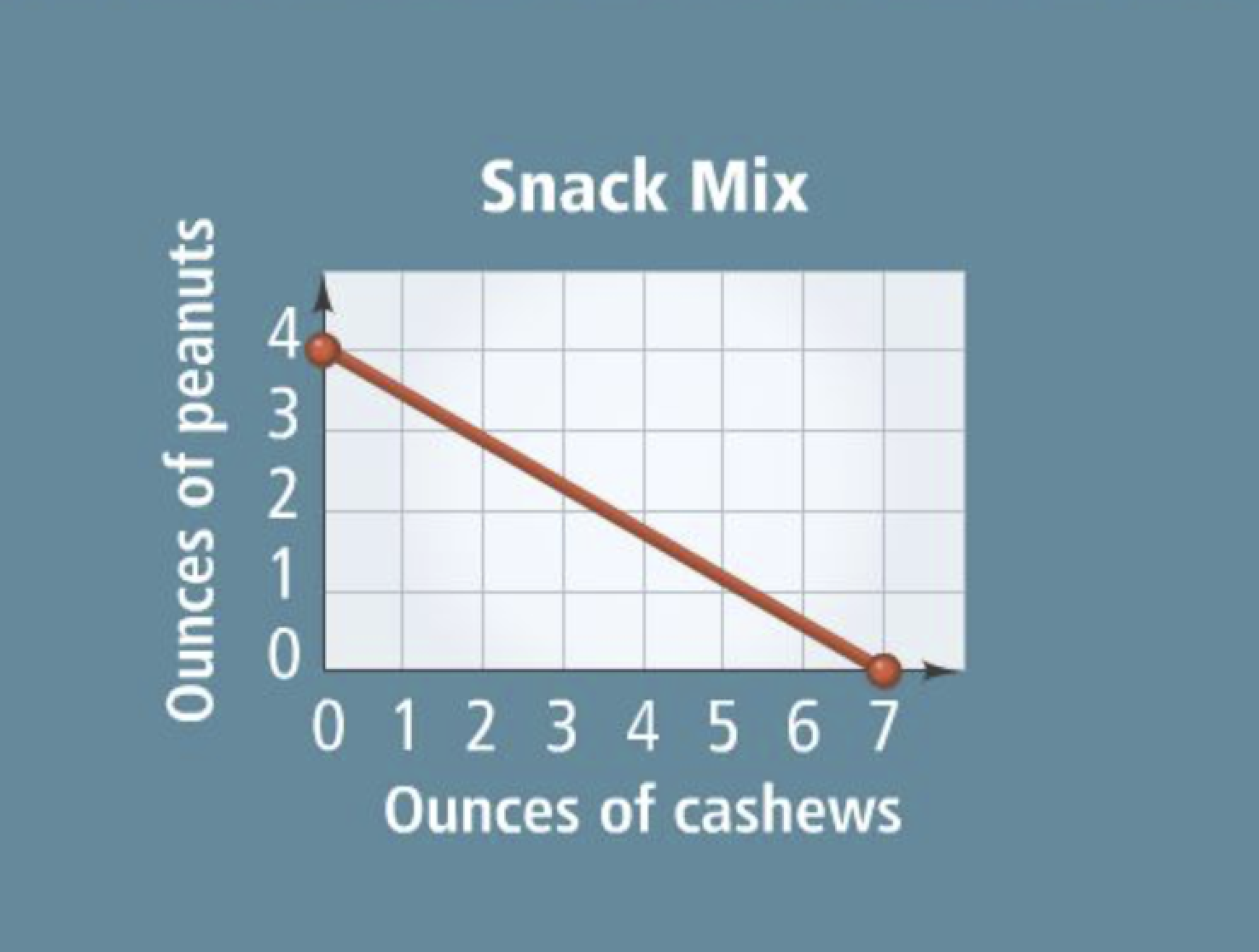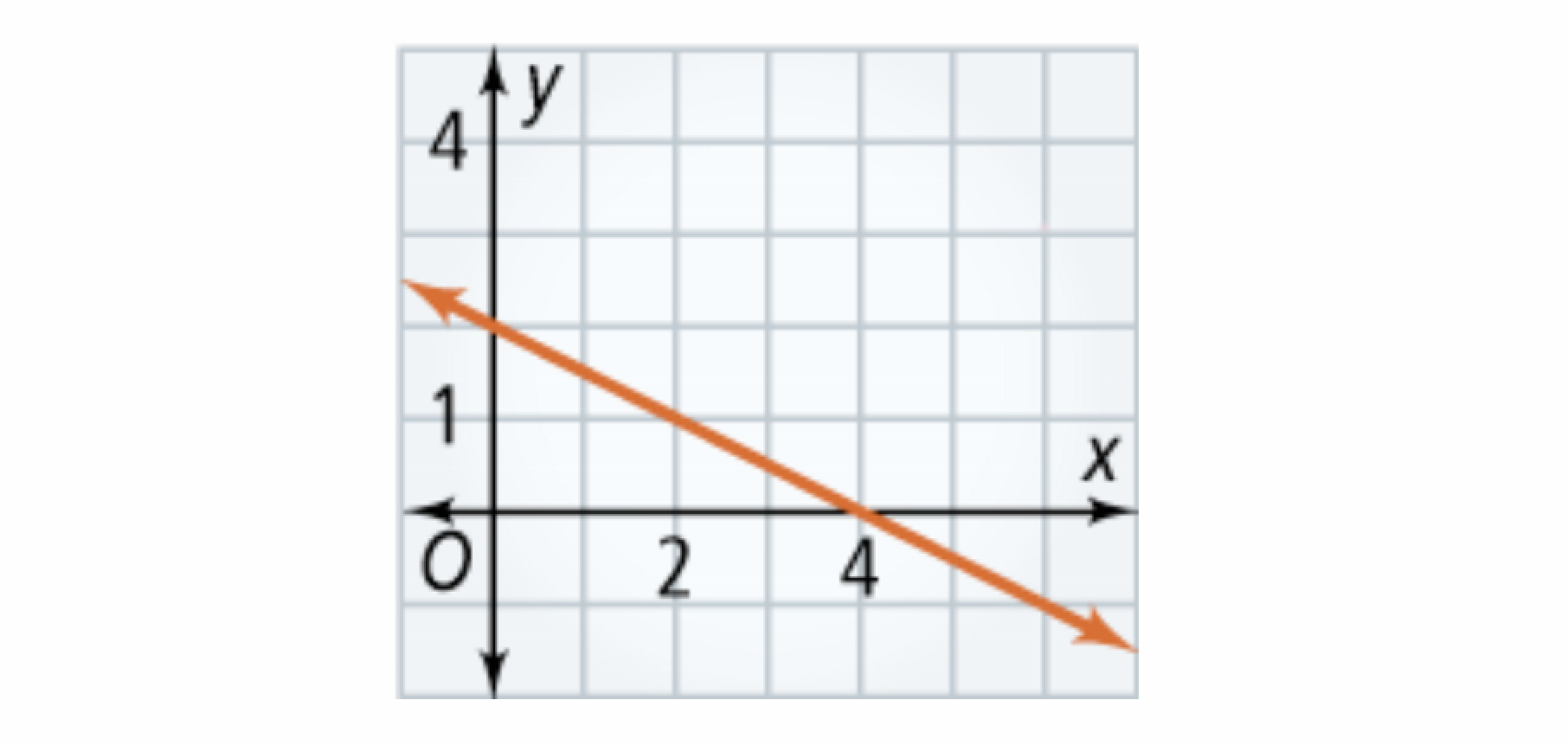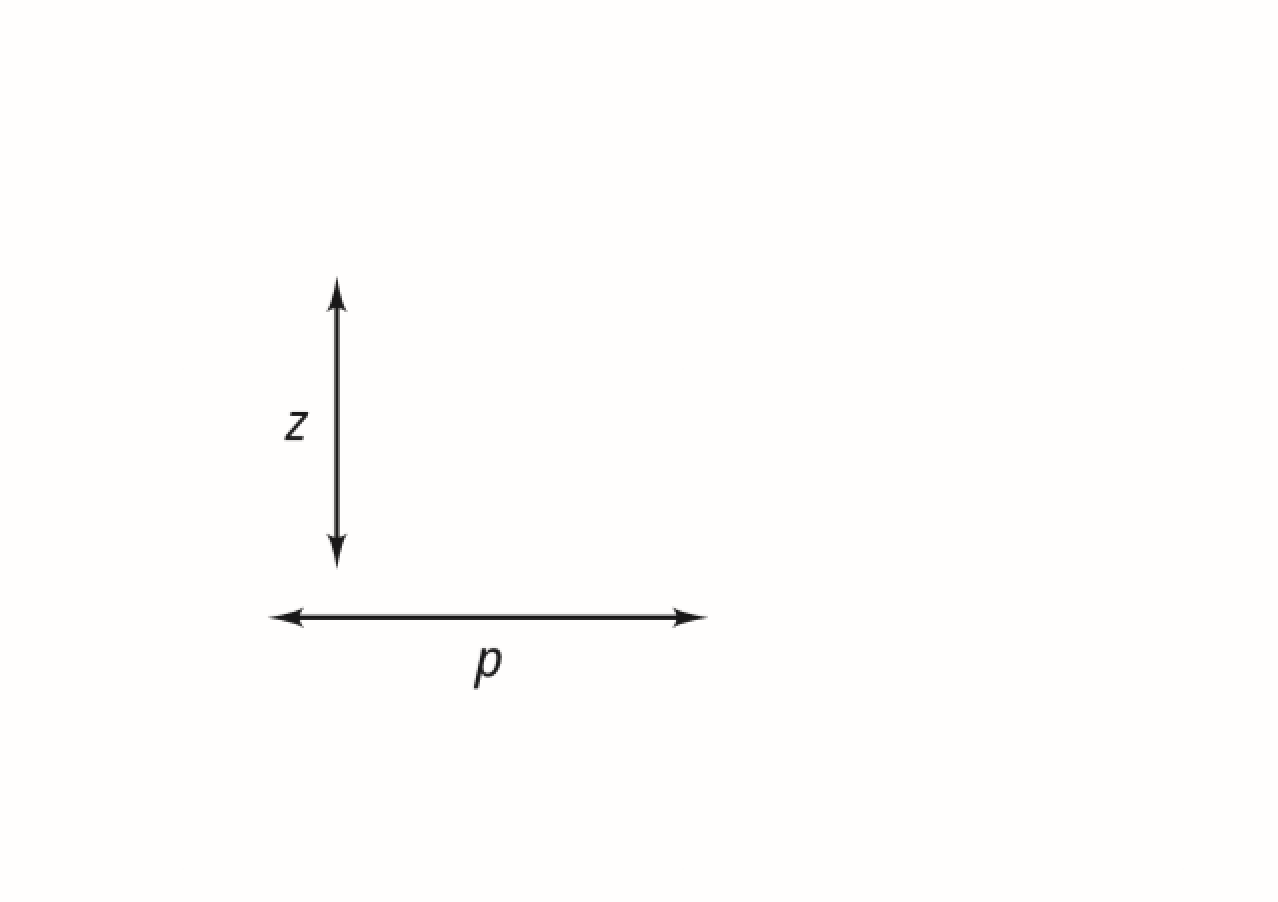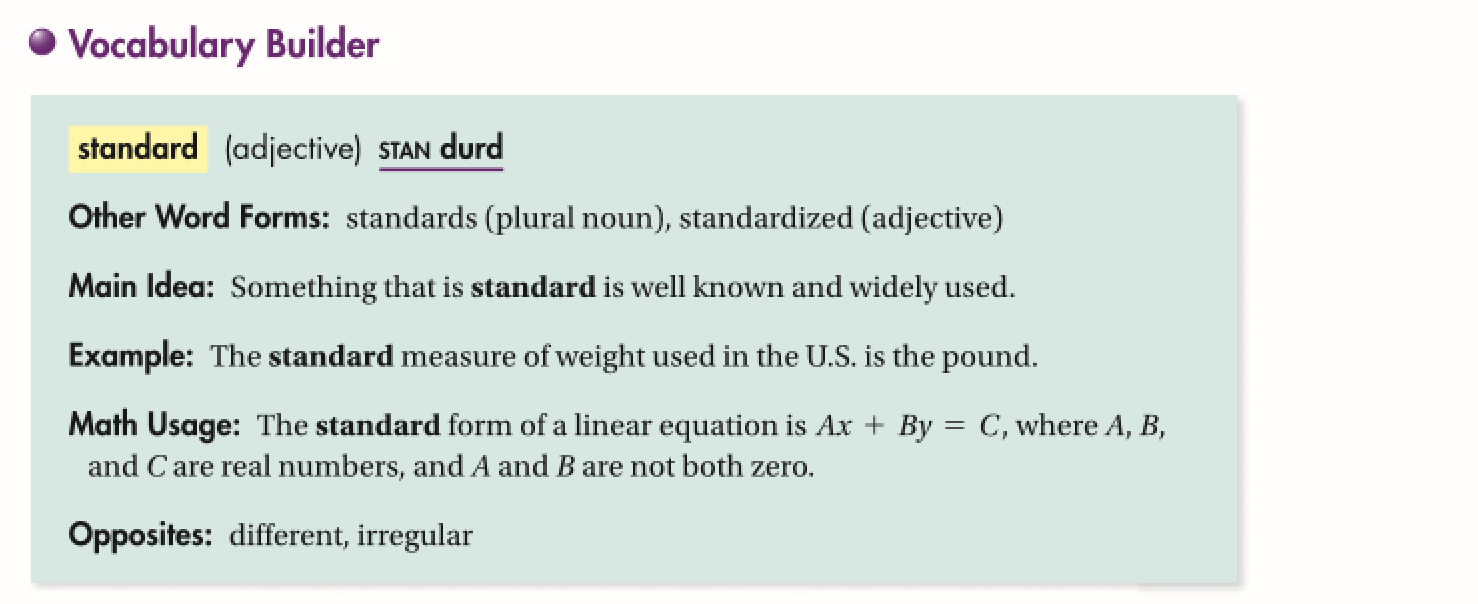Algebra 1 5-5 Complete Lesson: Standard Form
starstarstarstarstarstarstarstarstarstar
5 (1 rating)
by Matthew Richardson
| 32 Questions
Note from the author:
A complete formative lesson with embedded slideshow, mini lecture screencasts, checks for understanding, practice items, mixed review, and reflection. I create these assignments to supplement each lesson of Pearson's Common Core Edition Algebra 1, Algebra 2, and Geometry courses. See also mathquest.net and twitter.com/mathquestEDU.The outlined content above was added from outside of Formative.1
2
1
10 pts
Solve It! An athlete wants to make a snack of peanuts and cashews that will contain a certain amount of protein. Cashews have 4 g of protein per ounce and peanutes have 7 g of protein per ounce. Based on the graph, how many grams of protein will the athlete's mix contain?
14
0
36
28
2
10 pts
Solve It! What do the points (7, 0) and (0, 4) represent in the context of the scenario?3
3
10 pts
Problem 1 Got It?
A
B
C
D4
4
10 pts
Problem 1 Got It?
A
B
C
D
5
10 pts
Problem 2 Got It? What is the graph of the equation?
Be sure to include relevant graph detail: label axes, indicate units on both axes, and use arrows to represent end behavior, as appropriate.
6
10 pts
Problem 3 Got It? What is the graph of the equation?
Be sure to include relevant graph detail: label axes, indicate units on both axes, and use arrows to represent end behavior, as appropriate.
7
10 pts
Problem 3 Got It? What is the graph of the equation?
Be sure to include relevant graph detail: label axes, indicate units on both axes, and use arrows to represent end behavior, as appropriate.
8
10 pts
Problem 3 Got It? What is the graph of the equation?
Be sure to include relevant graph detail: label axes, indicate units on both axes, and use arrows to represent end behavior, as appropriate.
9
10 pts
Problem 3 Got It? What is the graph of the equation?
Be sure to include relevant graph detail: label axes, indicate units on both axes, and use arrows to represent end behavior, as appropriate.10
10
10 pts
Problem 4 Got It?
A
B
C
D11
12
11
10 pts
Problem 5 Got It? In Problem 5, suppose the store charged \$15 for each movie. What equation describes the numbers of songs and movies you can purchase for \$60?
15x + y = 60
x + 15y = 60
15x + 12y = 60
12
10 pts
Problem 5 Got It? Reasoning: What domain and range are reasonable for the equation in the previous item? Explain.13
10 pts
What are the x- and y-intercepts of the graph of the equation?

x-intercept: 0; y-intercept: -9/4
x-intercept: 3; y-intercept: 0
x-intercept: 3; y-intercept: -9/4
x-intercept: 0; y-intercept: 3
14
10 pts
What is the graph of the equation?
Be sure to include relevant graph detail: label axes, indicate units on both axes, and use arrows to represent end behavior, as appropriate.
15
10 pts
Is the graph of y = -0.5 a horizontal line, a vertical line, or neither?
Horizontal line
Vertical line
Neither
16
10 pts
What is the equation below written in standard form using integers?

x - 2y = -6
x - y = -6
x + 2y = 6
x + y = 3
17
10 pts
Gift Cards: A store sells gift cards in preset amounts. You can purchase gift cards for \$10 or \$25. You have spent \$285 on gift cards.

a. Write an equation in standard form to represent this situation.
b. What are three combinations of gift cards you could have purchased?18
5 pts
Vocabulary: Tell whether the linear equation is in slope-intercept form, point-slope form, or standard form.

Slope-intercept form
Point-slope form
Standard form
19
5 pts
Vocabulary: Tell whether the linear equation is in slope-intercept form, point-slope form, or standard form.

Slope-intercept form
Point-slope form
Standard form
20
5 pts
Vocabulary: Tell whether the linear equation is in slope-intercept form, point-slope form, or standard form.

Slope-intercept form
Point-slope form
Standard form
21
5 pts
Vocabulary: Tell whether the linear equation is in slope-intercept form, point-slope form, or standard form.

Slope-intercept form
Point-slope form
Standard form22
22
10 pts
Reasoning: Which form would you use to write an equation of the line graphed: slope-intercept form, point-slope form, or standard form? Explain.23
10 pts
Review Lessson 5-4: Match the equations in point-slope form and slope-intercept form with the pairs of points they contain.
• y = -(5/8)x + (17/8)
• y + 1 = x + 2
• y + 2 = (4/3)x
• y + 1 = -(5/8)(x - 5)
• y = (4/3)x - 2
• y = x + 1
• (5, -1), (-3, 4)
• (0, -2), (3, 2)
• (-2, -1), (1, 2)
24
10 pts
Review Lesson 3-6: Solve the compound inequality. Graph your solution.
Include relevant graph detail.
25
10 pts
Review Lesson 3-6: Solve the compound inequality. Graph your solution.
Include relevant graph detail.
26
10 pts
Review Lesson 3-6: Solve the compound inequality. Graph your solution.
Include relevant graph detail.
27
10 pts
Review Lesson 5-1: Match each slope with the pair of points it represents.
• m = 2
• m = 0
• m = 3
• (0, -4), (2, 0)
• (5, 5), (3, -1)
• (-4, 2), (5, 2)28
28
10 pts
Vocabulary Review: Match the correct word to complete each sentence.
• hoirzontal
• vertical
• Line z is a __?__ line.
• Line p is a __?__ line.
• A line with slope 0 is a __?__ line.
• A line with undefined slope is a __?__ line.29
10 pts
Use Your Vocabulary: Use the correct word to complete each sentence.
• standard
• standards
• standardized
• In gymnastics, judges use a set of __?__ to award a score.
• Most English words have a __?__ pronunciation.
• Many states use __?__ tests to assess their students' performance.
30
10 pts
Vocabulary Review: Identify the form of each equation.
• slope-intercept form
• point-slope form
• standard form
• y = -6x + 4
• 3x - 7y = 42
• y - 6 = 4(x - 2)
31
100 pts
Notes: Take a clear picture or screenshot of your Cornell notes for this lesson. Upload it to the canvas. Zoom and pan as needed.

For a refresher on the Cornell note-taking system, click here.
32
10 pts
Reflection: Math Success
Add to my formatives list

Formative uses cookies to allow us to better understand how the site is used. By continuing to use this site, you consent to the Terms of Service and Privacy Policy.